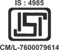An ISO 9001:2008 Certified Company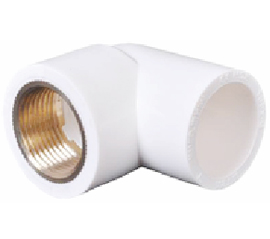#### brass elbow

Size Packing Rs.
1/2" 50x5=250 30.00
3/4" 35x5=175 60.00
1" 24x5=120 100.00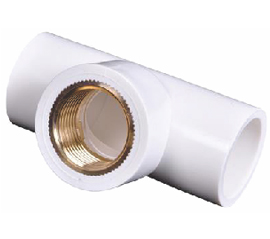#### brass tee

Size Packing Rs.
1/2" 36x5=180 36.00
3/4" 27x5=135 70.00
1" 18x5=90 120.00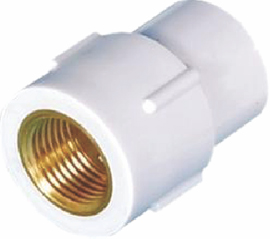#### brass F.T.A.

Size Packing Rs.
1/2" 72x5=360 28.00
3/4" 56x5=280 58.00
1" 36x5=180 90.00
1"1/4" 25x5=125 150.00
1"1/2" 20x4=80 200.00
2" 12x4=48 300.00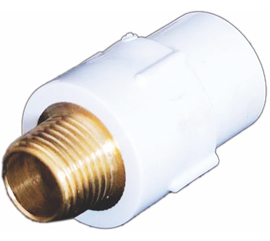#### brass M.T.A.

Size Packing Rs.
1/2" 72x5=360 45.00
3/4" 49x5=245 80.00
1" 30x5=150 110.00
1"1/4" 20x5=100 230.00
1"1/2" 20x5=100 300.00
2" 12x4=48 440.00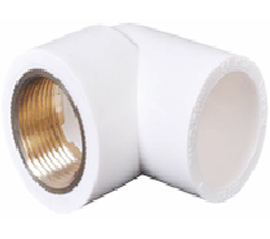#### reducer brass elbow

Size Packing Rs.
3/4"x1/2" 40x5=200 40.00
1"x1/2" 30x5=150 50.00
1"x3/4" 32x5=160 70.00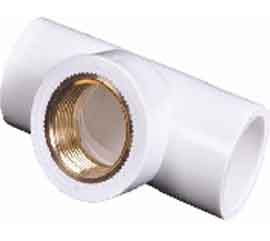#### reducer brass tee

Size Packing Rs.
3/4"x1/2" 30x5=150 50.00
1"x1/2" 21x5=105 60.00
1"x3/4" 21x5=105 90.00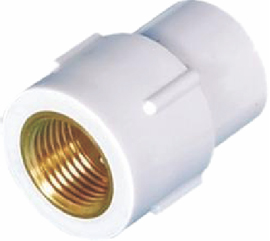#### reducer brass F.T.A.

Size Packing Rs.
3/4"x1/2" 56x5=280 40.00
1"x1/2" 49x5=245 50.00
1"x3/4" 49x5=245 70.00#### reducer brass M.T.A.

Size Packing Rs.
3/4"x1/2" 49x5=245 56.00
1"x1/2" 40x5=200 70.00
1"x3/4" 40x5=200 100.00